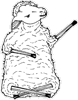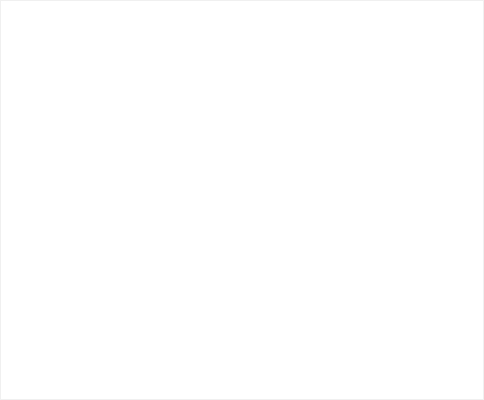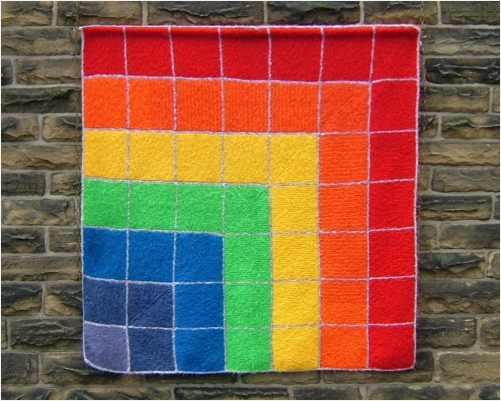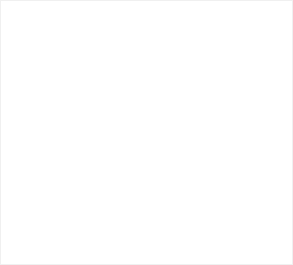﻿ Some Square Over The RainbowClick to read general information about afghansBuy nowOther places to visit

Order Form

Mental Blocks

The World of Illusion Knittingfor this afghan

on the order formBuy nowSOME SQUARE OVER THE RAINBOW

It is obvious that it has the seven colours of the rainbow and that it has squares but it also represents one way of generating square numbers.

Every square number is the sum of odd numbers. The odd numbers here are shown in the L shapes:

1 + 3 + 5 + 7 + 9 + 11 + 13 = 49, which is the square of 7.KNITTING INFORMATION

Use any yarn and needles of your choice. It is not essential to use seven different colours. The design works well in two colours. You don’t even need to have seven bands.

The afghan can be made to any size by adjusting the number, and width, of the squares.Some Square Over The RainbowRELATED DESIGNS

NUMBER AFGHANS

Counting Pane

Mere Bagatelle

Fibo-optic

Fibrenacci

Equal Parts

Double BaseCONSTRUCTION INFORMATION

The original afghan was constructed in L-shapes, using one yarn at a time. It is symmetrical about the diagonal.

The L-shapes are stitched together.

It could be knitted in strips.

Some Square Over The Rainbow has 49 small squares - 7 columns by 7 rows. It represents square numbers and one way of calculating them.

Each colour has an odd number of squares. There is one violet square, 3 indigo squares, 5 blue, 7 green, 9 yellow, 11 orange and 13 red, making the total of 49.

Square numbers are the sum of odd numbers.

The first square number is 1.

The second is 4 (2 x 2), which is 1 + 3.

The third is 9 (3 x 3), which is 1 + 3 + 5.

... and so on

The seventh is 1 + 3 + 5 + 7 + 9 + 11 + 13 = 49